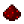# bit.tonumbFunction bit.tonumb Converts an array (numerically-indexed table) containing binary bit values to a number (the inverse of bit.tobits) Syntax bit.tonumb(table bit_tbl) Returns number the number resulting from the conversion of bit_tbl from binary Part of ComputerCraft API bitExample Convert the binary representation 10010 into its corresponding value, 18 Code ```t = {}t = 0t = 1t = 0t = 0t = 1print(bit.tonumb(t)) ``` Output 18